﻿ 空气污染与呼吸疾病的半参数统计分析 Semi-Parametric Statistical Analysis of Air Pollution and Respiratory Diseases

Statistics and Application
Vol.07 No.01(2018), Article ID:23701,7 pages
10.12677/SA.2018.71005

Semi-Parametric Statistical Analysis of Air Pollution and Respiratory Diseases

Yanyong Zhao1, Yuan Liu1, Hongxia Hao2, Zhiyang Yao1

1Institute of Statistics and Big Data, Nanjing Audit University, Nanjing Jiangsu

2School of Mathematics, Southeast University, Nanjing Jiangsu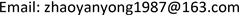Received: Jan. 17th, 2018; accepted: Jan. 31st, 2018; published: Feb. 7th, 2018ABSTRACT

The article mainly focuses on the relationship between the air pollution and diseases in respiratory system in Hong Kong based on the principal component dimensionality reduction method and partially linear models. In the empirical analysis, by comparing with the linear model and linear model with time, we find that the proposed method has a better predictive effect and the relationship between air pollution and respiratory diseases in Hong Kong is nonlinear.

Keywords:Air Pollution, Respiratory Diseases, Partially Linear Models

1南京审计大学，统计科学与大数据研究院，江苏 南京

2东南大学，数学学院，江苏 南京1. 引言

2. 模型的估计

$Y={X}^{\text{T}}\beta +g\left(T\right)+\epsilon$ (1)

$\stackrel{˜}{g}\left(t,\beta \right)=\underset{i=1}{\overset{n}{\sum }}{W}_{hi}\left(t\right)\left({y}_{i}-{x}_{i}^{\text{T}}\right)\beta$ (2)

$SS\left(\beta \right)=\underset{i=1}{\overset{n}{\sum }}{\left[{y}_{i}-{x}_{i}^{\text{T}}\beta -\stackrel{˜}{g}\left({t}_{i},\beta \right)\right]}^{2}$ (3)

${\stackrel{^}{\beta }}_{n}={\left({\stackrel{^}{X}}^{\text{T}}\stackrel{^}{X}\right)}^{-1}{\stackrel{^}{X}}^{\text{T}}\stackrel{^}{y}$ (4)

${\stackrel{^}{g}}_{n}\left(t\right)=\underset{i=1}{\overset{n}{\sum }}{W}_{hi}\left(t\right)\left({y}_{i}-{x}_{i}^{\text{T}}{\stackrel{^}{\beta }}_{n}\right)$ (5)

3. 空气污染与呼吸疾病数据的实证分析

$\text{MSE}=\frac{1}{n}\underset{i=1}{\overset{n}{\sum }}{\left({y}_{i}-{\stackrel{^}{y}}_{i}\right)}^{2}$$\text{MAE}=\frac{1}{n}\underset{i=1}{\overset{n}{\sum }}|{y}_{i}-{\stackrel{^}{y}}_{i}|$ (6)

$\begin{array}{l}{Z}_{1}=0.\text{123}{X}_{1}+0.\text{393}{X}_{2}+0.\text{321}{X}_{3}+0.\text{173}{X}_{4}-0.220{X}_{5}-0.288{X}_{6}\\ {Z}_{2}=0.\text{494}{X}_{1}-0.\text{007}{X}_{2}+0.\text{329}{X}_{3}-0.\text{483}{X}_{4}+0.082{X}_{5}+0.216{X}_{6}\\ {Z}_{3}=0.\text{414}{X}_{1}+0.\text{085}{X}_{2}-0.\text{171}{X}_{3}+0.304{X}_{4}+0.752{X}_{5}-0.288{X}_{6}\end{array}$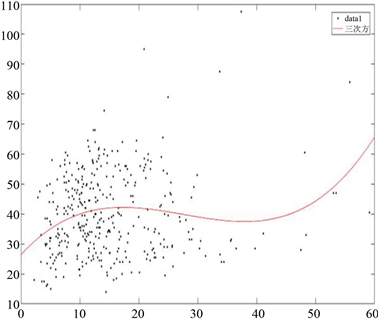Figure 1. Z1 versus daily number of hospitalized patients with respiratory disease (Y)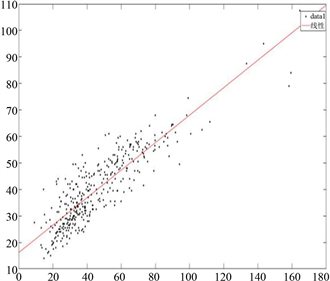Figure 2. Z2 versus daily number of hospitalized patients with respiratory disease (Y)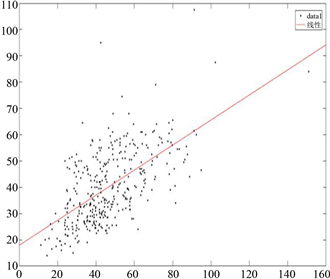Figure 3. Z3 versus daily number of hospitalized patients with respiratory disease (Y)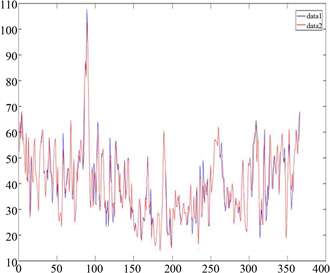Figure 4. True and estimated values for daily number of hospitalized patients with respiratory disease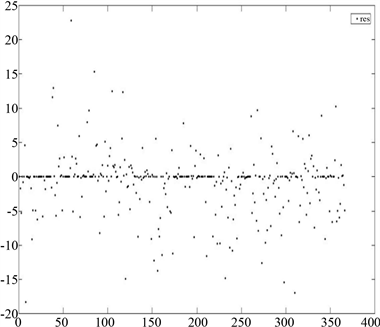Figure 5. Scatter graph of residuals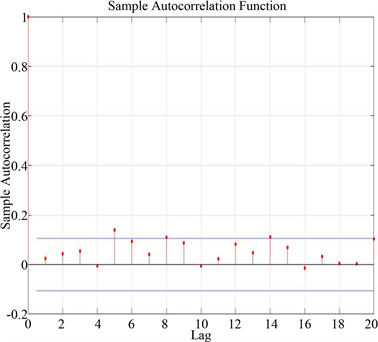Figure 6. Autocorrelation function graph of residualsTable 2. MSE and MAE of daily number of hospitalized patients with respiratory disease

MAE。通过表2可知，利用主成分降维方法和部分线性模型预测的结果明显比另外两种模型的预测更准确。

4. 结论

Semi-Parametric Statistical Analysis of Air Pollution and Respiratory Diseases[J]. 统计学与应用, 2018, 07(01): 32-38. http://dx.doi.org/10.12677/SA.2018.71005

1. 1. Engle, R.F., Granger, W.J., Rice, J. and Weiss, A. (1986) Serniparametric Estimates of the Relation between Weather and Elec-tricity Sales. Journal of the American Statistical Association, 80, 310-319. https://doi.org/10.1080/01621459.1986.10478274

2. 2. Cuzick, J. (1992) Semiparametric Additive Regression. Journal of the Royal Statistical Society, Series B, 54, 831-843.

3. 3. 梁华, 黄四民. 用半参数部分线性模型分析居民消费结构[J]. 数量经济技术经济研究, 1994(10): 33-35.

4. 4. Schmalensee, R. and Stoker, T.M. (1999) Household Gasoline Demand in the United States. Econometrica, 67, 645-662. https://doi.org/10.1111/1468-0262.00041

5. 5. Liang, H., Hardle, W. and Sommerfeld, V. (2000) Bootstrap Approximation in a Partially Linear Regression Model. Journal of Statistical Planning and Inference, 91, 413-426. https://doi.org/10.1016/S0378-3758(00)00191-9

6. 6. Ma, Y.Y., Chiou, J.M. and Wang, N. (2006) Efficient Semiparametric Estimator for Heteroscedastic Partially Linear Models. Biometrika, 93, 75-84. https://doi.org/10.1093/biomet/93.1.75

7. 7. You, J. and Chen, G. (2007) On Inference for a Semiparametric Partially Linear Regression Model with Serially Correlated Errors. The Canadian Journal of Statistics, 35, 515-531. https://doi.org/10.1002/cjs.5550350404

8. 8. 李启华, 蓝志青, 等. 基于半参数估计的笔记本电脑特征价格指数研究[J]. 东北财经大学, 2011(2): 84-85.

9. 9. Jiang, Y. (2015) Robust Estimation in Partially Linear Regression Models. Journal of Applied Statistics, 42, 2497-2508. https://doi.org/10.1080/02664763.2015.1043862

10. 10. 杨宜平, 薛留根, 王学娟. 高维部分线性模型中的变量选择[J]. 北京工业大学学报, 2011, 37(2): 291-295.

11. 11. Luo, J. and Gerard, P. (2013) Using Thresholding Difference-Based Estimators for Variable Selection in Partial Linear Models. Statistics and Probability Letters, 83, 2601-2606. https://doi.org/10.1016/j.spl.2013.08.011